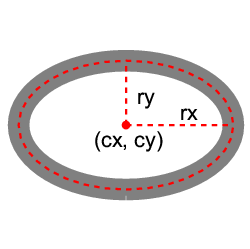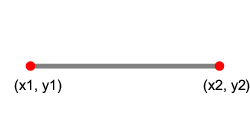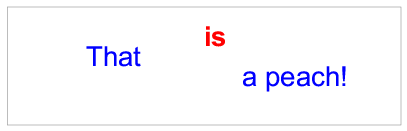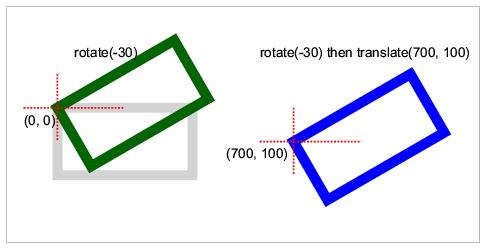# Scalable Vector Graphics (SVG)

SVG can be included in any XML document simply by adding a `svg` element.

The `display`, `float`, and `flow` properties and all `margin`, `padding`, `border`, and `background` properties can be applied to the top-level `svg` element but not to child elements within it.

The following elements are supported inside the `svg` element:

 `g` for grouping related graphics elements `rect` for drawing rectangles `circle` for drawing circles `ellipse` for drawing ellipses `line` for drawing lines `polyline` for drawing polylines `polygon` for drawing polygons `path` for drawing arbitrary paths `text` for drawing text `tspan` for adjusting text and font properties inside the `text` element `image` for including bitmap image files `a` for creating hyperlinks

## Viewbox and viewport

The `svg` element should include view box and view port information so that the SVG content can be correctly mapped to the containing box.

Attributes
`width` the width of the viewport
(100% of the container width, if omitted)
`height` the height of the viewport
(100% of the container width, if omitted)
`viewBox`
(x y width height)
the x, y coordinate of the top left corner of the viewbox and the width and height of the viewbox

XML

```<svg width="3cm" height="2cm" viewBox="0 0 300 200">
<!-- the SVG content -->
</svg>
```

## Rectangles

Rectangles are created using the `rect` element.

Attributes
`x`, `y` the x, y coordinates of the top left corner of the rectangle
`width` the width of the rectangle
`height` the height of the rectangle
`rx`, `ry` the radii for rounded corners

XML

```<svg viewBox="0 0 500 300"
width="10cm" height="6cm">
<g fill="none" stroke="gray" stroke-width="20">
<rect x="50" y="50" width="400" height="200"
rx="80" ry="50"/>
</g>
</svg>
```

Output## Circles

Circles are created using the `circle` element.

Attributes
`cx`, `cy` the centre of the circle
`r` the radius of the circle

XML

```<svg viewBox="0 0 200 200"
width="5cm" height="5cm">
<g fill="none" stroke="gray" stroke-width="20">
<circle cx="100" cy="100" r="100"/>
</g>
</svg>
```

Output## Ellipses

Ellipses are created using the `ellipse` element.

Attributes
`cx`, `cy` the centre of the ellipse
`rx`, `ry` the radii of the ellipse

XML

```<svg viewBox="-10 0 220 200"
width="6cm" height="6cm">
<g fill="none" stroke="gray" stroke-width="20">
<ellipse cx="100" cy="100" rx="100" ry="60" />
</g>
</svg>
```

Output## Lines

Lines are created using the `line` element.

Attributes
`x1`, `y1` the start point of the line
`x2`, `y2` the end point of the line

XML

```<svg viewBox="0 0 100 50"
width="6cm" height="3cm">
<g stroke="gray" stroke-width="2">
<line x1="10" y1="25" x2="90" y2="25"/>
</g>
</svg>
```

Output## Polylines

Polylines are created using the `polyline` element.

Attributes
`points` list of points that defines the line. Points are separated by whitespace and each point is a pair of x, y coordinates separated by comma.

XML

```<svg viewBox="0 0 600 400"
width="6cm" height="4cm">
<g stroke="gray" stroke-width="10">
<polyline points="50,300 200,300 200,100
400,100 400,300 550,300" />
</g>
</svg>
```

Output## Polygons

Polygons are created using the `polygon` element.

Attributes
`points` list of points that defines the line. Points are separated by whitespace and each point is a pair of x, y coordinates separated by comma.

XML

```<svg viewBox="600 0 600 400"
width="6cm" height="4cm">
<g fill="none" stroke="gray" stroke-width="10">
<polygon points="850,75  958,137.5 958,262.5
850,325 742,262.6 742,137.5" />
</g>
</svg>
```

Output## Paths

The `path` element is for drawing arbitrary paths. The `d` attribute inside the element `path` takes a list of path commands. Parameters of a command can be separated either by whitespace or a comma.

Command Parameters Meaning
M (absolute)
m (relative)
(x y)+ moves to the given (x, y) coordinates to start a new subpath
Z or z none closes the current subpath
L (absolute)
l (relative)
(x y)+ draws a line to the given (x, y) coordinates
H (absolute)
h (relative)
x+ draws a horizontal line to the given x coordinates
V (absolute)
v (relative)
y+ draws a vertical line to the given y coordinates
C (absolute)
c (relative)
(x1 y1 x2 y2 x y)+ draws a cubic Bézier curve to the given (x,y) coordinates, uses the given (x1,y1) coordinates as the first control point, the (x2,y2) coordinates as the second control point
S (absolute)
s (relative)
(x2 y2 x y)+ short hand of the C/c command: the reflection relative to the current point of the second control point of the previous command is used as the first control point.
Q (absolute)
q (relative)
(x1 y1 x y)+ draws a quadratic cubic Bézier curve to the given (x,y) coordinates, uses the given (x1,y1) coordinates as the control point.
T (absolute)
t (relative)
(x y)+ short hand of the Q/q command: the reflection relative to the current point of the control point of the previous command is used as the control point.
A (absolute)
a (relative)
(rx ry x-axis-rotation large-arc-flag sweep-flag x y)+ draws an elliptical arc to (x, y): the size and rotation of the ellipse are defined by two radii (rx, ry) and the x-axis-rotation; the center (cx, cy) of the ellipse is also determined by the large-arc-flag and sweep-flag constraint.

XML

```<svg viewBox="0 0 600 400"
width="12cm" height="8cm">
<g fill="none" stroke="red" stroke-width="10">
<path d="M100,200 C100,100 250,100
250,200 S400,300 400,200" />
</g>
</svg>
```

Output## Text

The `text` element is for writing arbitrary text. The `tspan` element is supported inside the `text` element, for adjusting text position and font properties.

Attributes
`x``y` the absolute position of the text
`dx``dy` the relative offset of the current text position (optional)

XML

```<svg viewBox="0 0 1000 300"
width="10cm" height="3cm">
<text x="200" y="150" fill="blue" font-size="70">
That
<tspan dx="2em" dy="-50" font-weight="bold" fill="red" >
is
</tspan>
<tspan dy="100">
a peach!
</tspan>
</text>
</svg>
```

Output## Images

The `image` element is for including external image files, either bitmap images or other SVG images.

Attributes
`xlink:href` the URL of the image to include
`x`, `y` the x, y coordinates of the top left corner of the image
`width` the width of the image
`height` the height of the image

Note that the `href` element must be placed in the XLink namespace: `http://www.w3.org/1999/xlink` in order to work.

XML

```<svg xmlns:xlink="http://www.w3.org/1999/xlink"
...
<image x="100" y="100" width="50" height="50" xlink:href="myimage.jpg"/>
...
</svg>
```

The `a` element is for creating hyperlinks.

Attributes
`xlink:href` the URL of the link

Note that the `href` element must be placed in the XLink namespace: `http://www.w3.org/1999/xlink` in order to work.

XML

```<svg xmlns:xlink="http://www.w3.org/1999/xlink"
...
...
</a>
...
</svg>
```

## Transformations

The `transform` attribute can be used on `g`, `path`, and all of the basic shape elements. It accepts any sequence of the following transformations separated by whitespace.

• translate(tx ty)
• scale(sx [sy])
• rotate(angle)
• skewX(angle)
• skewY(angle)
• matrix(a b c d e f)

XML

```<svg viewBox="-150 -200 1400 700" width="12cm" height="6cm">
<g fill="none" stroke-width="30">
<rect width="400" height="200" stroke="lightgray"/>
<rect width="400" height="200" stroke="darkgreen" transform="rotate(-30)"/>
<g transform="translate(700 100) rotate(-30)">
<rect width="400" height="200" stroke="blue"/>
</g>
</g>
</svg>
```

Output## Style Properties

The following style properties are supported on SVG elements:

• `font-family`
• `font-size`
• `font-style`
• `font-weight`
• `fill`
• `fill-opacity`
• `fill-rule`
• `marker-start`
• `marker-mid`
• `marker-end`
• `stop-color`
• `stroke`
• `stroke-dasharray`
• `stroke-dashoffset`
• `stroke-linecap`
• `stroke-linejoin`
• `stroke-opacity`
• `stroke-width`
• `text-anchor`

Style properties can be applied using SVG presentation attributes:

```<rect fill="yellow" stroke="blue" stroke-width="20"
width="200" height="100"/>
```

Or by using CSS properties inside the `style` attribute or element:

```<rect style="fill:yellow; stroke:blue; stroke-width:20"
width="200" height="100"/>
```